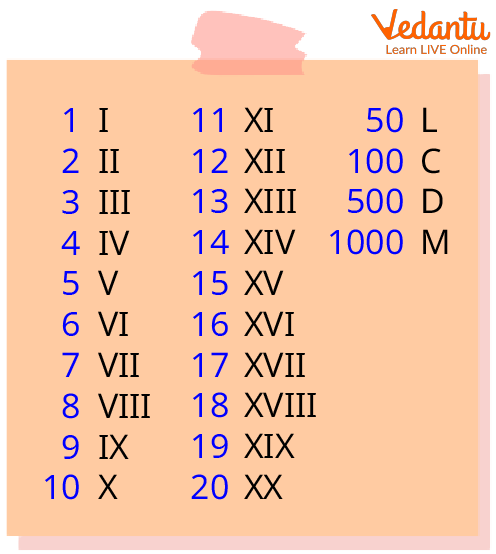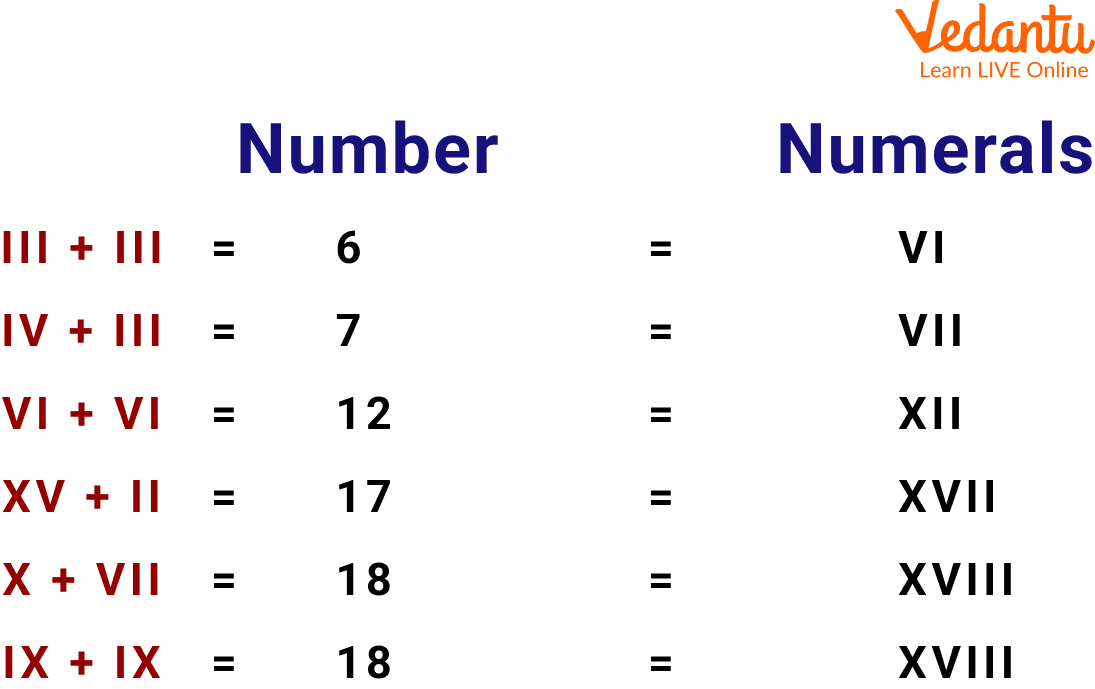Courses
Courses for Kids
Free study material
Offline Centres
MoreLast updated date: 29th Nov 2023
Total views: 114k
Views today: 1.14k## Introduction of Roman Number Addition

Have you ever seen alphabetical letters to represent the number? Like I, V, L, M, and D and if your answer is yes then these are the Roman numbers. In earlier times Romans used these symbols to denote numbers. But still, you can see the use of Roman numerals in your everyday life. But if you don't know anything about Roman numerals then don't worry. In this article, we are going to discuss the Roman number, Roman number rules, Roman number addition and maths rules for addition and multiplication. So let's get started.

## What are Roman Numerals?

Roman numerals are a type of writing system that dates back to ancient Rome. It was the usual way of writing numbers in Europe during the Late Middle Ages and also throughout the Roman Empire. The letters used in this system are from the Latin alphabet. Modern styles use seven symbols, each with a fixed integer value.Roman Number

Roman Empire's fall but Roman numerals were still in use. Roman numbers started to be gradually replaced by Arabic numerals starting in the 14th century, however, some applications still use Roman numerals today.

Roman numerals are essentially a decimal and "base ten" number system, but instead of place value notation the system uses a set of symbols with fixed values, including "built-in" powers of ten.

## Roman Numbers Rules

Roman numbers follow some rules, which help resolve how the number is written. It will also help us in reading Roman numbers.

• If a Roman number is repeated, its value is added as many times as it occurs.

For example, III=3, XXX=30 etc.

• The numbers V, L and D are never repeated.

• If a smaller number is written to the right of a number with a greater value, then its value gets added to the value of the greater number.

For example, VII=5+2=7, XII=12 and so on.

• If a smaller number is written to the left of a number with a greater value, then its value gets subtracted from the value of the greater number.

• A number is not repeated more than three times.

• The numbers V, L and D are never written to the left of several greater values i.e. V, L and D are never subtracted.

• The number I can be subtracted from V and X only. The number X can be subtracted from L, M, and C only.

## How to Convert Roman Numerals into Numbers?

We can convert CXV to a decimal number. Each Roman numeral represents a number. C, X, and V represent 100, 10, and 5, respectively. Now all the numbers are added, CXV = C + X + V = 100 + 10 + 5 = 115. The Roman numeral CXV represents 115.

Points that should be remembered in order to convert Roman numeral into numbers:

• When any number is written after a larger number, it needs to be added to the larger number.

For example, VIII is written as 5 + 3 = 8.

• When any number is written before a larger number, it needs to be subtracted from the larger number.

For example, IV is written as 5 - 1 = 4.

## Method of Addition of Roman Numerals

Roman numerals are written in addition and subtractive notation. Addition notation means that the individual values in a Roman numeral are added together to get the total value. In the case of VII, you add up the values for V and two I's. V is 5 and I is 1, so 5 + 1 + 1 = 7.The subtractive notation means that the value of the Roman numeral is found by taking the last roman number and subtracting the value of the character that comes just before it. In the case of XL, you subtract the value of X from the value of L. X is 10 and L is 50, so 50-10 = 40.

## Solved Examples

Example 1: Convert the number 549 into the Roman numeral form.

Ans: The number 549 can be written as 549 = 500 + 40 + 9. As 40 and 9 do not have a symbol, hence, 40 is written as: (50 - 10) and 9 is written as: (10 - 1). Now 549 is expressed as 549 = 500 + (50 - 10) + (10 - 1).

The Roman numeral for each number is 500, 40, and 9. These are denoted by the letters D, XL, and IX, respectively. Thus, the Roman numeral for 549 is:

549 = D + (XL) + (IX) = DXLIX

Thus 549 is expressed as DXLIX as Roman numerals.

Example 2: Convert MCCLXI into a decimal number

Ans: The Roman numeral MCCLXI can be split as:

MCCLXI = M + C + C + L + X + I = 1000 + 100 + 100 + 50 + 10 + 1 = 1261

Thus, MCCLXI is expressed as 1261 in decimal numbers.

Example 3: Subtract XI from XLV. Write the answer in Roman numerals.

Ans: As XI = 10 + 1 = 11 and XLV = 50 - 10 + 5 = 45

Now, XLV – XI

= 45 – 11

= 34

We write 34 in Roman numerals as XXXIV.

Therefore, XLV - XI equals XXXIV.

## Practice Questions

A. Add and subtract the given Roman numerals

Q 1. XLVI - XXVIII.

Ans. XVIII (18)

Q 2. LXXXVI - LIX.

Ans. XXVII (27)

Q 3. LVII - XXIV.

Ans. XXXIII (33)

Q 4. LXIV - XLIV.

Ans. XX (20)

Q 5. LVII + XXXIV.

Ans. XCI (91)

Q 6. XXIV + LVI.

Ans. LXXX (80)

## Summary

So in this article, we learned about so many interesting things which were related to Roman numerals but we were not aware of that. We also cleared our concepts in a very enjoyable way. Here, we learned about everything related to Roman numerals like the addition of Roman numerals, Roman number rules, and many other interesting things. But the main thing which we need to keep in mind while learning math is to learn it with fun and if we learn something then even those topics which seem difficult to us like math rules for addition and multiplication will also become easy to us just like Roman numerals.

## FAQs on Roman Numbers Addition

1. Where are Roman numerals used in everyday life?

Roman numerals are often used in books for denoting chapter numbers, fancy clocks and watches.

2. Is there a zero in Roman numerals?

No.

3. What are the drawbacks of using Roman numerals?

Roman numeral has many disadvantages including:

• There is no symbol for 0.

• Fractions can not be represented using Roman numerals.

• Not easy to understand and calculate by common people.